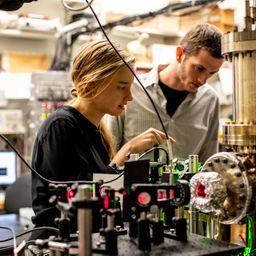## a. 2.90 \times 10^{-19} Jb. 2.90 \times 10^{-19} Jc. 1.65 \times 10^{26} \mathrm{W}d. 5.69 \times 10^{44} \text { photons } / s

Quantum Physics

Atomic Physics

### Discussion

You must be signed in to discuss.
##### Top Physics 103 EducatorsLB##### Jared E.

University of Winnipeg### Video Transcript

Hey, soon this problem. We're told that a star has a radius of 8.5 times 10 of the eight, and we want what else we told on the peak wavelength of 6 85 So Lamda is 6 85 centimeters, and we want to get, um, the energy of a photons with that wavelength. All right. We can just use, um, ia's h half and then, um, f a c over lambda. So he is HC over lambda I h is Planck's constant C is the speed of lion and lamb. Bust this Lambert right here. So I'm gonna go ahead and plug that into a calculator. 6.626 times. 10 to the minus. 34 times 2.98 meat times 10 to the eighties. All divided by 6 85 times 10 to the minus nine. And so with that, I got, um, So is this right? H time? See, Beehive. Um, I got 2.8996 many other digits. Times 10 to the minus 19. Jules. So I will go ahead and write that out. So 2.90 let's see. 3662.90 times. 10 to the minus 19 Trolls. Mr. Wilczek, that TJ and we want to get the surface temperature of the star. So we can you figure that out using mines Displacement law. So for that, what we want to do is say that Lambda Times T is equal to point to a 98 times 10 to the minus two Calvin meters, and we want to take both sides and divided by Lambda to get the temperature. So I'll just go ahead and erase that so that we can get that the temperature is equal to this. And, um, I'm gonna go ahead and put that into a calculator. My period. I need a sense of the minus two, right? Bye. We have links, and then I got 4230 Calvin, and I'm sorry. I'm impossible. Quick. Okay, I'm in now. We want to get the, um, read. That energy is omitted from the star. And so I eat. We want to get the power. And so we want to borrow some of the formulas from chapter 11. So that tells us that power is equal to sig my e, the service area of the star Times t to the fourth. And so, um, this Sigma C is equal to have to pull the chapter again. Um, 5.6696 times 10 to the minus eight in Mississippi is one and a s a surface area. So that's gonna be pi r squared. So cig my e pi r squared. If you're not sure where this comes from, just review the chapter, Um, Chapter 11. Or you could just google. Um, Stefan saw. So if I go ahead and plug all of this into a calculator, let's see what I got and I'll pause. Why didn't I do that? Calculation? Okay, so that I got 1.65 times 10 to the 26 wants. And great. So let's see what else they want in this question. And then d we don't want to get the rate that photons leave the surface of the star. So, um, so this is the energy of a foe. Time of a particular photons on. And then this is the total amount of power energy per unit time. And so, um, so let's answer the question. Maybe like in one second. Um, how many photons have left. So after one second, we know that, um, this much energy, this number let me write. Sir. Let me write down what I'm thinking. Who? I really don't want to use another page, but I won't lose all these numbers, some kind of box, everything up and then make use of my spare space. So? So, after one second, we know that, um, 1.65 times 10 to the 26 jewels have been used. And then each photon has this many jewels. Um, so you can kind of say times once you do. Sort of a unit conversion. One photo time has 2.90 times. 10 to the minus 19 drawls. And then that's how you can get how many photons are emitted per second. So let's go ahead and divide out. These numbers will take the last number and divide it by two point. I know it was 10. The my ass 19. And so without, I got 5.68 times 10 to the 44. Sorry, I should be a four, um, photons. So that would be the number of protons. And I'll go ahead on label this little section DUniversity of Washington

#### Topics

Quantum Physics

Atomic Physics

##### Top Physics 103 EducatorsLB##### Jared E.

University of Winnipeg